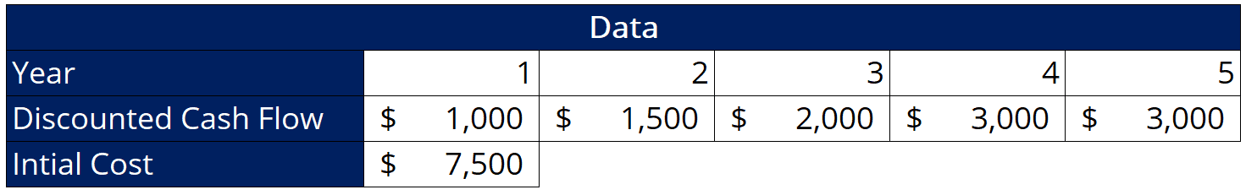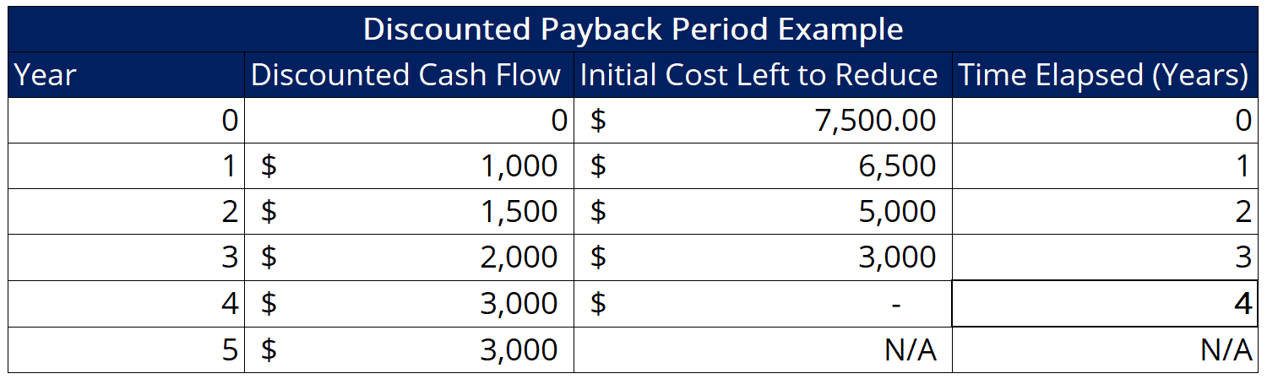# Discounted Payback Period

Calculates the amount of time that it will take for a project to "break even"

## What is the Discounted Payback Period?

The discounted payback period is a modified version of the payback period that accounts for the time value of money. Both metrics are used to calculate the amount of time that it will take for a project to “break even”, or to get the point where the net cash flows generated cover the initial cost of the project. Both the payback period and the discounted payback period can be used to evaluate the profitability and feasibility of a specific project.Other metrics, such as the internal rate of return (IRR), profitability index (PI), net present value (NPV), and effective annual annuity (EAA) can be used to quantify the profitability of a given project. To make the best decision about whether to pursue a project or not, a company’s management needs to decide which metrics to prioritize. Management then looks at a variety of metrics in order to obtain complete information. Usually, companies are deciding between multiple possible projects. Comparing various profitability metrics for all projects is key to making a well-informed decision.

### Discounted Payback Period Formula

There are two steps involved in calculating the discounted payback period. First, we must discount (i.e., bring to the present value) the net cash flows that will occur during each year of the project.

Second, we must subtract the discounted cash flows from the initial cost figure in order to obtain the discounted payback period. Once we’ve calculated the discounted cash flows for each period of the project, we can subtract them from the initial cost figure until we arrive at zero.

### Discounted Payback Period Example

Assume a business that is considering a given project. Below are some selected data from the discounted cash flow model created by the company’s financial analysts:As we can see here, the project returns a positive discounted cash flow in its first year and sees its yearly discounted cash flow grow to \$3,000 in later years. We also learn that the project cost is \$7,500. Using the given information, we can calculate the discounted payback period as follows:In this case, we see that the project’s payback period is 4 years. Since the project’s life is calculated at 5 years, we can infer that the project returns a positive NPV. Thus, the project will likely add value to the business if pursued.

### Payback Periods

One observation to make from the example above is that the discounted payback period of the project is reached exactly at the end of a year. Obviously, that may not always be the case. In other circumstances, we may see projects where the payback occurs during, rather than at the end of, a given year.

In such situations, we will first take the difference between the year-end cash flow and the initial cost left to reduce. Next, we divide the number by the year-end cash flow in order to get the percentage of the time period left over after the project has been paid back.

The next step is to subtract the number from 1 to obtain the percent of the year at which the project is paid back. Finally, we proceed to convert the percentage in months (e.g., 25% would be 3 months, etc.), and add the figure to the last year in order to arrive at the final discounted payback period number.

We hope you enjoyed reading CFI’s explanation of the Discounted Payback Period. CFI offers the Financial Modeling & Valuation Analyst (FMVA)™ certification program for those looking to take their careers to the next level. To keep learning and advancing your career, the following resources will be helpful:

• Adjusted Present Value (APV)
• Forecasting Methods
• Weighted Average Cost of Capital (WACC)
• Valuation Methods

### Financial Analyst Training

Get world-class financial training with CFI’s online certified financial analyst training program!

Gain the confidence you need to move up the ladder in a high powered corporate finance career path.

Learn financial modeling and valuation in Excel the easy way, with step-by-step training.